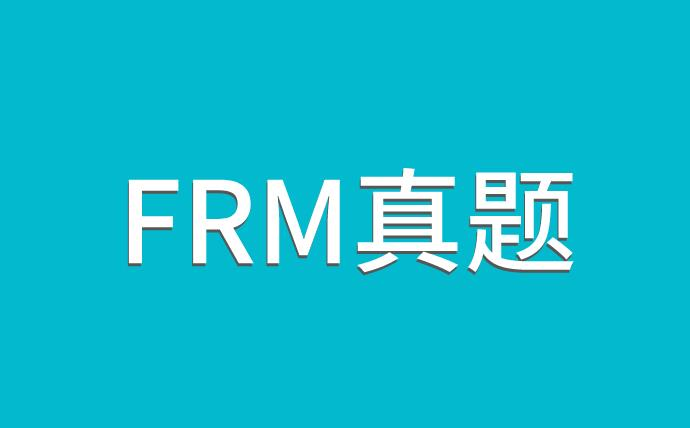2021年新版FRM备考资料下载
• 考纲对比
• 学习计划
• 思维导图
• 复习资料
• 历年真题
• 词典及公式FRM考试都是选择题的形式，考生在备考中一定要认真备考。近日，有考生咨询FRM真题解析哪里有？重要吗？

FRM真题在备考中对于考生是很重要的，尤其是冲刺阶段，一定要做大量的真题。下面是小编列举的相关真题解析，希望对你有所帮助！》》》戳：各科视频讲义+历年真题+21年原版书（PDF版）免·费领取

An analyst is looking at various models used to incorporate drift into term structure models. The Ho-Lee Model:

A) Incorporates no-risk premium to the interest rate model allowing rates to vary according to their volatility.

B) Incorporates drift as a premium to interest rates that remains constant over time.

C) Allows for a risk premium to be applied to interest rates that changes over time.

D) Incorporates drift into the model following the assumption that rates revert to the long-run equilibrium value.

Arisk manager is constructing a term structure model and intends to use the Cox-Ingersoll-Roll Model. Which of the following describes this model?A) The model presumes that the volatility of the short rate will increase at a predetermined rate.

B) The model presumes that the volatility of the short rate will decline exponentially to a constant level.

C) The model presumes that the basis-point volatility of the short rate will be proportional to the rate.【资料下载】[融跃财经]FRM一级ya题-pdf版

D) The model presumes that the basis-point volatility of the short rate will be proportional to the square root of the rate.